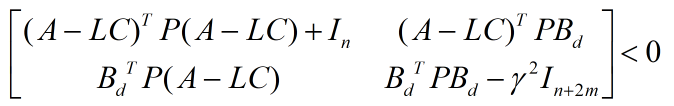# Applying schur complement

Hello, My question is as follows:
Let a matrixbe a negative definite with P is a symmetric positive definite matrix and I want to optimize gamma in order to find the optimal value of L.
So How can I apply schur complement on this matrix ?
Thanks

I don’t know what your decision variables are, but how do you expect this problem to be feasible? How can`(A-L*C)'*P*(A-L*C)+eye(n)` possibly be negative definite (which it needs to be to make the SDP negative definite) when P is positive definite?